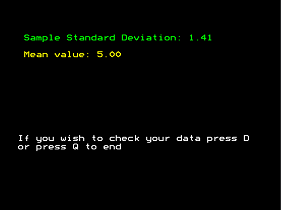STATISTICAL TOOLS
 Published by: AVP Computing Produced by: AVP Computing Subject: Mathematics Age Range: 16 - 18 Format: 5.25 in 40 Track DFS disc Compatibility: BBC Model B Original Price: Unknown First released: Unknown Protection: AVP 1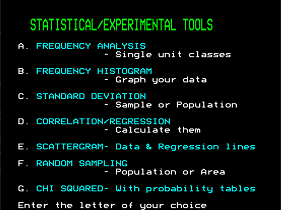Notes

Contains a number of programs for the statistical analysis of experimental data.

 Program: Chi-Squared Language: BASIC Programmer: Unknown Genre: Mathematics Notes: Calculates the chi-squared values of data input by the user.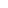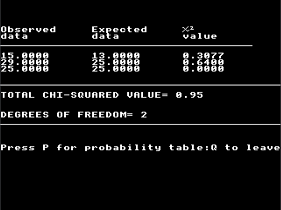Program: Correlation/Regression Language: BASIC Programmer: Unknown Genre: Mathematics Notes: Calculates the correlation coefficient of data input by the user and then will calculate values for data input by the user based on the regression analysis of the data..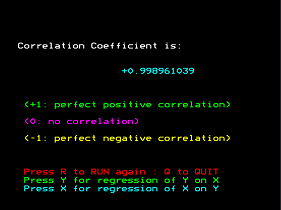Program: Frequency Analysis Language: BASIC Programmer: Unknown Genre: Mathematics Notes: Performs a frequency analysis of data input by the user.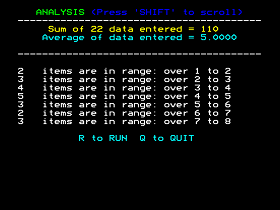Program: Frequency Histogram Language: BASIC Programmer: Unknown Genre: Mathematics Notes: Produces a bar chart of the frequency analysis of data input by the user.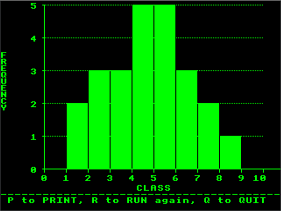Program: Random Sampling Language: BASIC Programmer: Unknown Genre: Mathematics Notes: Calculates the samples needed to be collected from an area or population whose parameters are entered by the user.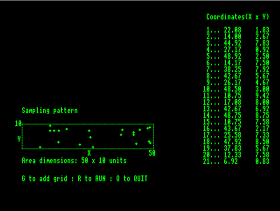Program: Scattergram Language: BASIC Programmer: Unknown Genre: Mathematics Notes: Produces a scattergram of data input by the user and shows the regression line of the data.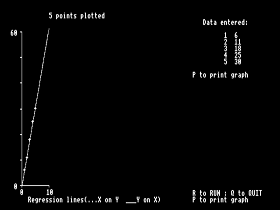Program: Standard Deviation Language: BASIC Programmer: Unknown Genre: Mathematics Notes: Calculates either the sample or population standard deviations of data input by the user.